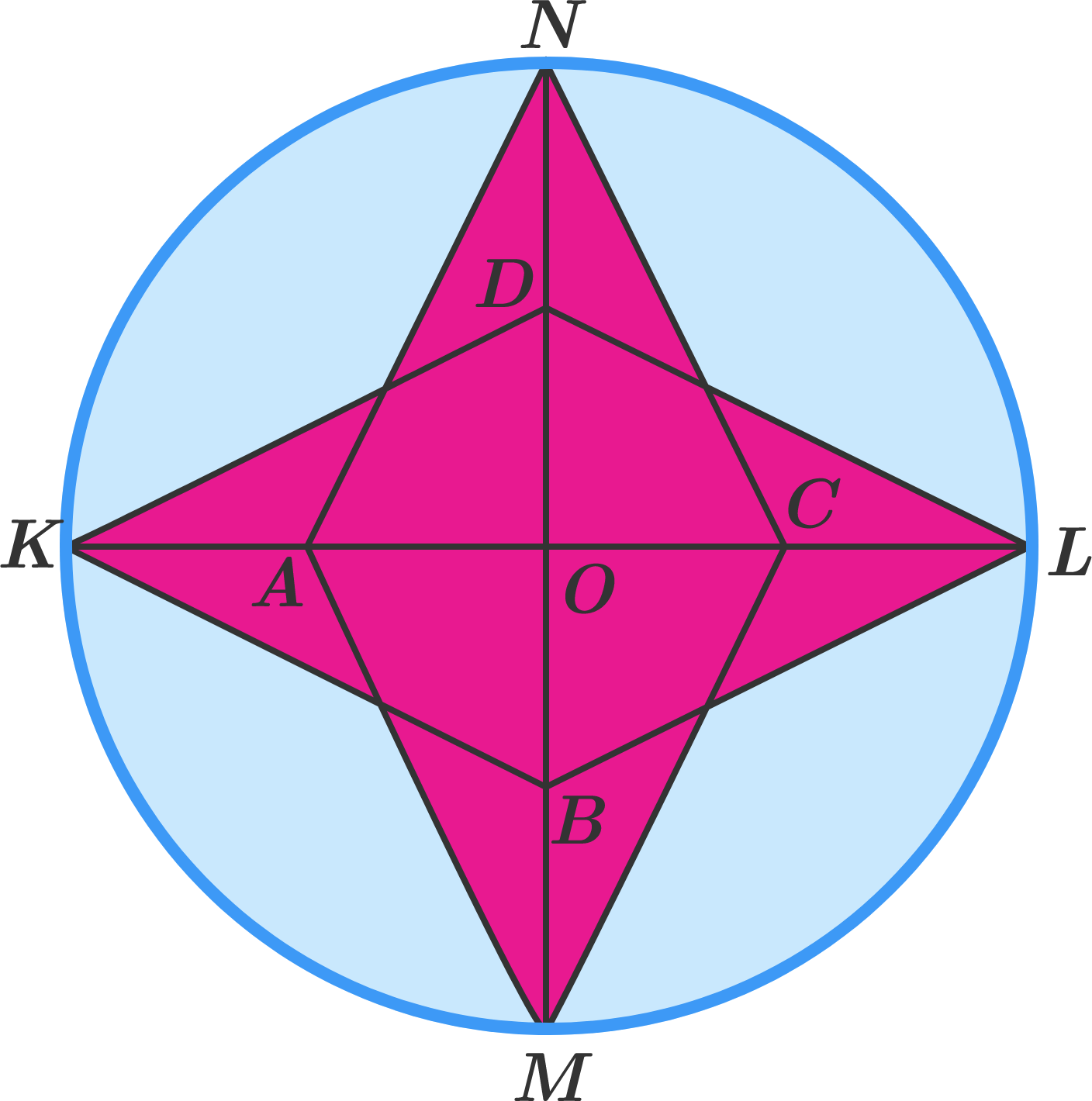# Star

Geometry Level 3

The circle with center $O$ in the figure has a radius of 6. Diameters $KL$ and $MN$ are perpendicular, and $A, B, C, D$ are the midpoints of $KO, MO, LO, NO,$ respectively.

What is the pink area?×